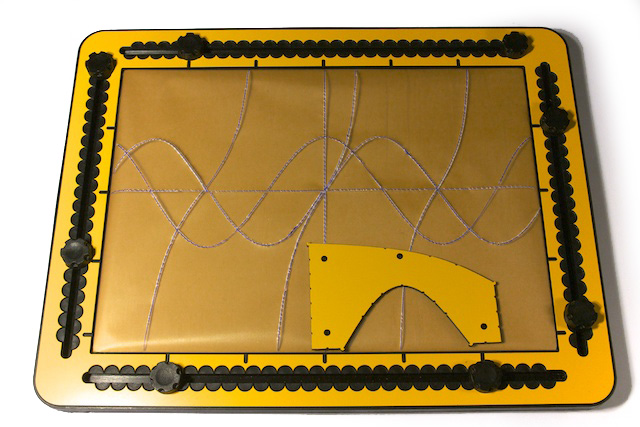The trigonometry tool supplied with the GraphGrid is used to draw basic trigonometric functions. The tool includes two curves: a half period of a sine wave and (almost) a quarter period of a tangent. The scale of both curves is the same. A distance of 4 centimeters along the X-axis corresponds with 90 degrees. When the sine and the tangent functions have a value of 1, the distance along the Y-axis in the drawing is 4 centimeters. These dimensions provide sufficient ‘tactile space’.